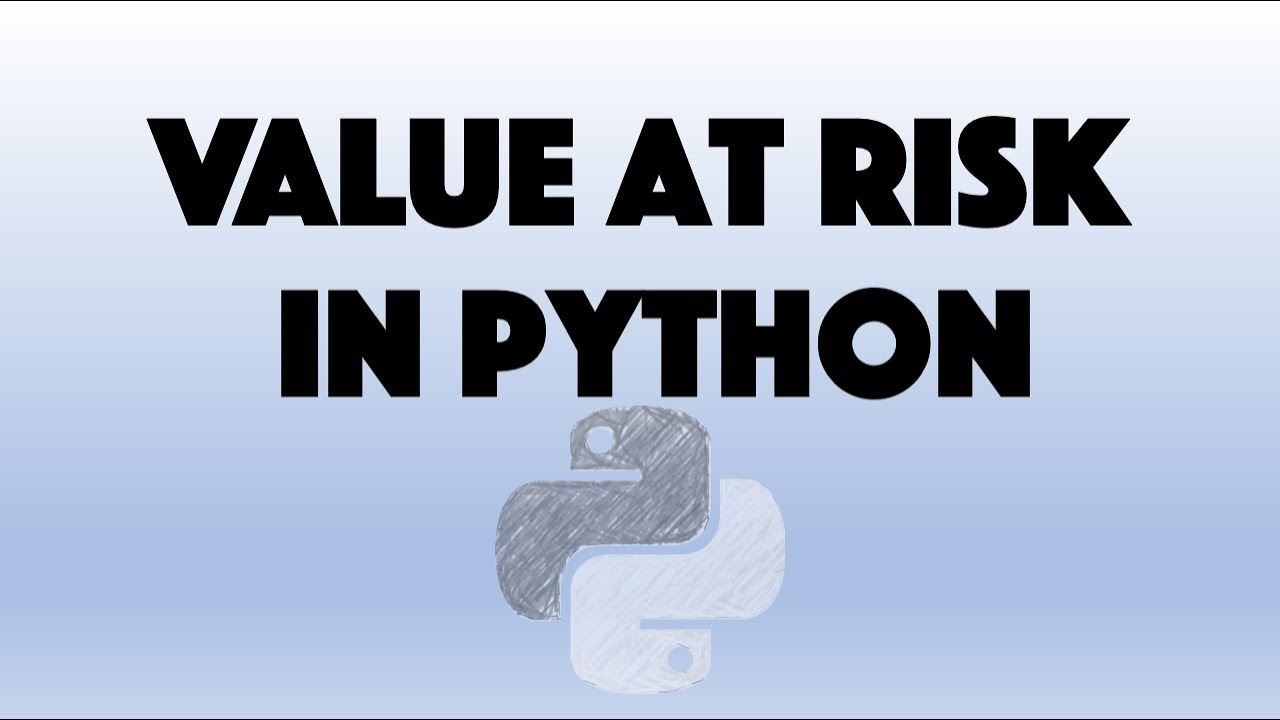# Value At Risk [VaR] and VaR Of A Portfolio in PythonValue At Risk [VaR] and VaR Of A Portfolio in Python. This video is showing how to calculate the Value at risk (VaR) of a portfolio containing multiple assets. The Value at risk is an important risk measure and is used in finance / banking , risk and Data Science related fields.

This video is showing how to calculate the Value at risk (VaR) of a portfolio containing multiple assets. The Value at risk is an important risk measure and is used in finance / banking , risk and Data Science related fields.

It starts with explaining how the Value at risk is calculated in Python, then applies this calculation to a two asset portfolio and then uses the covariance variance method to calculate the Value at risk again to make it scalable.

In the end I am pulling real stock prices to calculate the Value at Risk of a stock portfolio.

This video is not explaining the concept of the Value at risk in general and is just a technical implementation using Python. The method I am using is the so called Variance-Covariance-Method.

https://www.investopedia.com/terms/v/var.asp

If you have any questions please feel free to drop me a comment.

My playlist Python for finance where you find stuff like pulling free stock prices and calculating returns and many more:

https://www.investopedia.com/terms/p/portfolio-variance.asp

Very good resource with a different way of calculation but a very good explanation of the vector matrix multiplication:

https://financetrain.com/analytical-approach-to-calculating-var-variance-covariance-method/

#Python #Finance #VaR #ValueatRisk #Risk

## top 30 Python Tips and Tricks for Beginners

In this post, we'll learn top 30 Python Tips and Tricks for Beginners